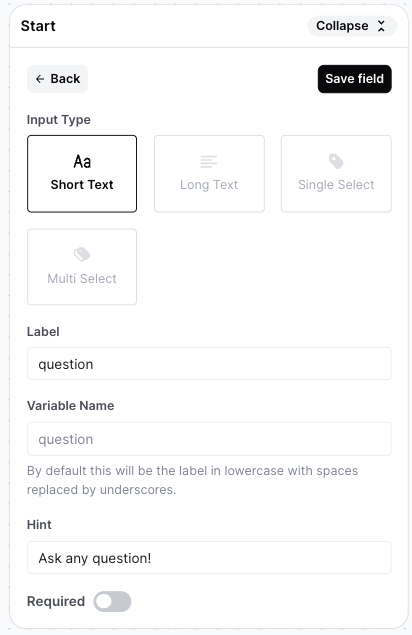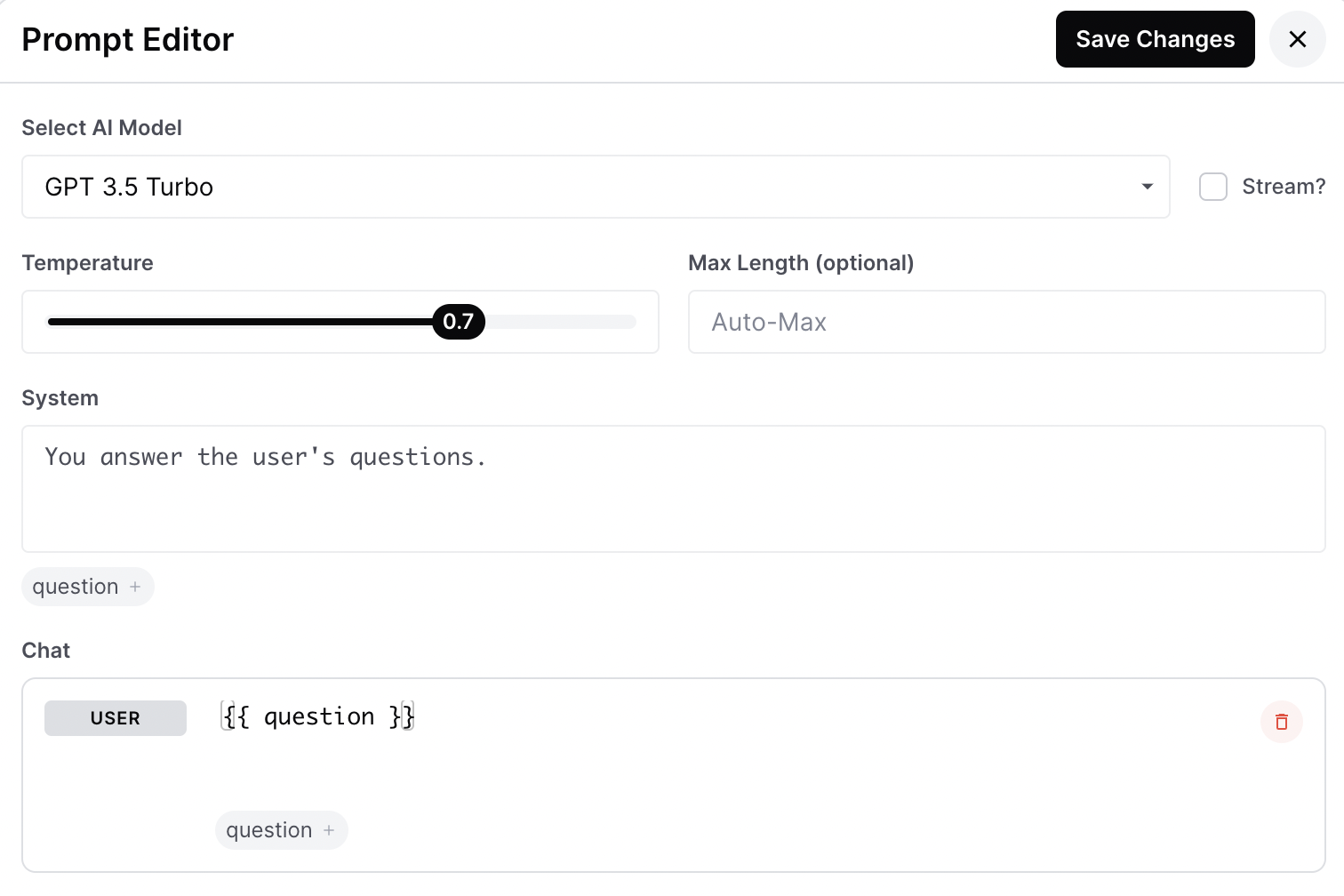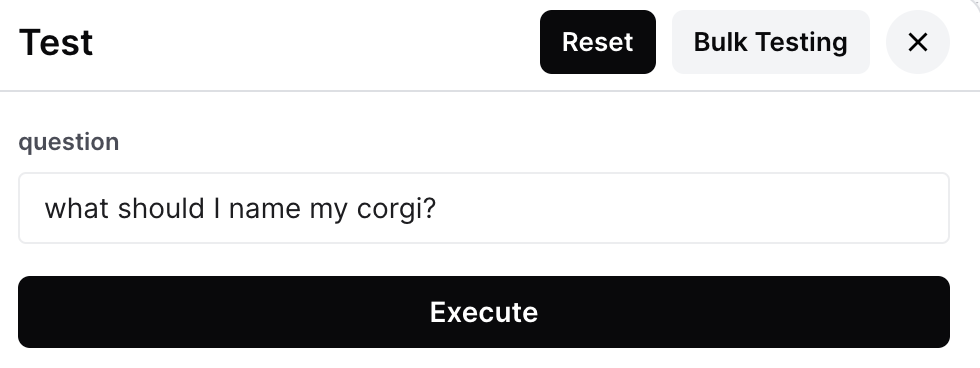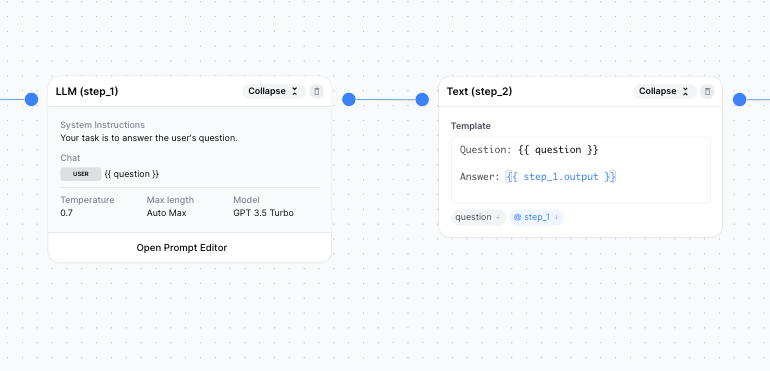# How to Reference Variables

Learn about Liquid syntax, the template syntax that allows you to reference variables in your Apps

App steps in AirOps utilize Liquid syntax to reference variable inputs and outputs, with the exception of code steps - Liquid syntax does not apply to code steps . These variables are either inputs defined at the "Start" step or outputs from previous steps.

## Syntax to Reference Variables by App Step

To access the inputs and step outputs, you will need to use the proper syntax depending on the step:

StepSyntax"Start" Step InputStep OutputJSON Step Output
LLMLiquid{{ my_input }}{{ step_x.output }}{{ step_x.output.key }}
TextLiquid{{ my_input }}{{ step_x.output }}{{ step_x.output.key }}
PythonPythonmy_inputstep_x["output"]step_x["output"]["key"]
JavascriptJavascriptmy_inputstep_x.outputstep_x.output.key

## Liquid Syntax Guide

### How to Reference Inputs in Liquid

1. App inputs are configured in the "Start" step - they can be used in any subsequent step. The following example creates the variable named question.1. To reference this input variable in an LLM or text step, you can simply type {{ question }} or click on the small tag below the step!1. Now, once you test this app, your input will replace {{ question }} in the step. In the following example, {{ question }} would be replaced by "what should I name my corgi?"### How to Reference an Output in Liquid

Just like with inputs, you can reference the outputs of previous steps in any subsequent step.

Currently, step names are automatically defined: step_1, step_2, so on and so forth. To reference the output of a step, simply type {{ step_1.output }} (where 1 is the number of the step)."What is the output exactly?" you might ask. Great question! In AirOps, you can view the exact output of a step in the Test panel (note: you can only view the output of the final step in a workflow).

In our example, "what should I name my corgi?", {{ step_1.output }} would be the result from GPT as shown here:

``````Size of an array